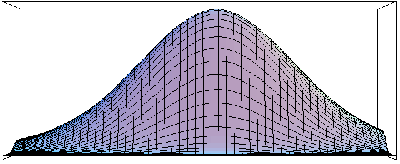### SIP - MATHEMATICA-Package for Statistical Inference

SIP makes it easy for the user to do classical likelihood based statistical inference. It contains procedures for maximum likelihood estimation, likelihood ratio tests of general hypotheses concerning parameters, and profile likelihood based confidence intervals for general interest functions of parameters.

SIP contains large collection of discrete and absolutely continuous univariate distributions and also multivariate distributions. It gives user possibility to form complicated models from the simpler ones.

SIP contains many sophisticated statistical models such as univariate/multivariate linear/non-linear regression model, logistic regression models, Poisson regression models, multinomial regression models etc.

SIP uses a new method for calculation of profile likelihood based confidence intervals for general parameter functions of interest in general parametric statistical models.SIP gives in addition to the statistical analysis procedures easy access to the powerful tools in for doing mathematics, graphics, programming, and presentation.

### Target audience

SIP has been developed mainly to assist applied statisticians in all subject areas to make inferences on real valued parameter functions of interest. It is also meant for teachers of statistical methods and inference in all subject areas and levels to be used for preparing courses and as tool in teaching statistical inference. Finally students from high schools to universities may use it to study statistical inference with it's easy user interface, wide ranging models, and large documentation.